# Simple interest And Compound interest for IBPS , SBI , RBI | Quantitative Aptitude | Quiz-4

## Simple interest And Compound interest for IBPS , SBI , RBI: Quiz 4

Simple interest And Compound interest plays a significant role in Quantitative Aptitude Section of banking exams such as IBPS, SBI and RBI PO and Clerk. You will get at least 4-5 questions from Simple interest And Compound interest in one of IBPS, SBI and RBI PO & clerk exam. So, aspirants should focus on Simple interest And Compound interest questions in detail. Here, we are providing you with the Simple interest And Compound interest questions quiz with the detailed solution so that you can easily prepare for Simple interest And Compound interest questions. We are providing here all-important latest pattern-based questions and Previous Year Questions of Simple interest And Compound interest of various Government Exam like IBPS, SBI, and RBI PO and Clerk exam. This Simple interest And Compound interest quiz we are providing is free. Attempt this Simple interest And Compound interest quiz to practice important questions with answers and solutions. And score better in IBPS, SBI and RBI PO and Clerk exam.

Simple interest And Compound interest Quiz to improve your Quantitative Aptitude for SBI Po & SBI clerk exam IBPS PO Reasoning , IBPS Clerk Reasoning , IBPS RRB Reasoning, LIC AAO ,LIC Assistant  and other competitive exam

1. Simple interest on a certain sum at the rate of 12% per annum for 3 years is Rs. 3024. What is the compound interest at the same sum at the rate of 20% for two years?

(a) Rs. 3850

(b) Rs 3696

(c) Rs 3966

(d) Rs 3669

(e) None of these2.The difference between C.I. and S.I. on a sum of Rs. 4800 at a certain rate for two years is Rs. 192. What is the rate of interest?

(a) 15%

(b) 20%

(c) 25%

(d) 10%

(e) None of these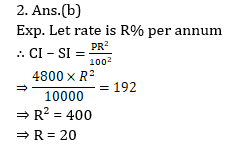3. What will be the compound interest on a sum of 62500 at the rate of 20% per annum for three years?

(a) 42,500

(b) 40,500

(c) 54,500

(d) 45,500

(e) None of these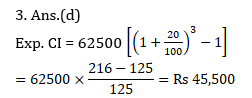4. A person has a total sum Rs. 16,650. He invests some part of this amount in scheme A at a rate of 10% for two years and rest part in scheme B at a rate of 20% for two years. The simple interest earned from both the scheme are equal. How much sum he invested in scheme B ?

(a) Rs. 5,650

(b) Rs. 6,550

(c) Rs. 6,650

(d) Rs. 5,550

(e) None of these5. The compound interest for two years on a certain sum is equal to the simple interest acrued on the same sum at the rate of 23% for three years. What is the rate of compound interest?

(a) 25%

(b) 25%

(c) 30%

(d) 35%

(e) None of these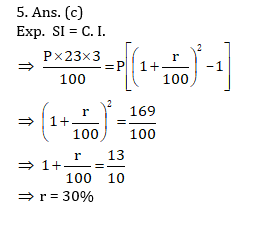6. If Rs. 12,000 is divided into two parts such that the simple interest on the first part for 3 years at 12% per annum is equal to the simple interest on the second part for years at 16% per annum, the greater part is :

(a) Rs. 8,000

(b) Rs. 6,000

(c) Rs. 7,000

(d) Rs. 7,500

(e) None of these7. Find the compound interest on Rs. 18,750 in 2 years the rate of interest being 4% for the first year and 8% for the second year.

(a) 2310

(b) 1130

(c) 3120

(d) None of these

(e) Can’t be determined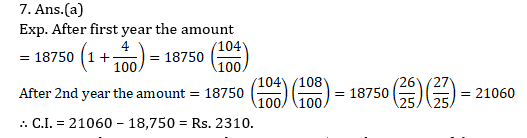8. At a simple interest Rs. 800 becomes Rs. 956 in three years. If the interest rate is increased by 3%, how much would Rs. 800 become in three years?

(a) Rs. 1020.80

(b) Rs. 1004

(c) Rs. 1028

(e) None of these9. A sum of money becomes eight times in 3 years if the rate is compounded annually. In how much time the same amount at the same compound interest rate will become sixteen times ?

(a) 6 years

(b) 4 years

(c) 8 years

(d) 5 years

(e) None of these10. Simple interest on a certain sum at 7% for 4 years is Rs. 3584. What will be the compound interest on the same principal at 4% in two years?

(a) Rs. 1054.48

(b) Rs. 1044.48

(c) Rs. 938

(d) Rs. 1064.84

(e) Rs. 1349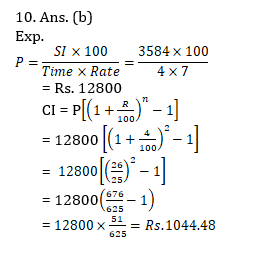#### Attempt Quantitative Aptitude Topic Wise Online Test Series

Recommended PDF’s for:

### Preparation Kit PDF

#### Most important PDF’s for Bank, SSC, Railway and Other Government Exam : Download PDF Now

AATMA-NIRBHAR Series- Static GK/Awareness Practice Ebook PDF Get PDF here
The Banking Awareness 500 MCQs E-book| Bilingual (Hindi + English) Get PDF here
AATMA-NIRBHAR Series- Banking Awareness Practice Ebook PDF Get PDF here
Computer Awareness Capsule 2.O Get PDF here
AATMA-NIRBHAR Series Quantitative Aptitude Topic-Wise PDF 2020 Get PDF here
Memory Based Puzzle E-book | 2016-19 Exams Covered Get PDF here
Caselet Data Interpretation 200 Questions Get PDF here
Puzzle & Seating Arrangement E-Book for BANK PO MAINS (Vol-1) Get PDF here
ARITHMETIC DATA INTERPRETATION 2.O E-book Get PDF here
3